# What are implied odds in sports betting

One of the most crucial parts of online betting is recognizing what the odds represent. Bookies set prices depending on the possibility of an event happening. All of them hide a percentage behind, known as implied probability. The process of turning the odds into percentages helps you reveal how much a bookmaker charges to let you place a bet. It’s challenging to make precise predictions in sports betting, so understanding the meaning of implied odds and identifying an outcome’s chances is undoubtedly beneficial. Read on to learn how these numbers work and the method you can calculate them while wagering. Also, discover how to convert the prominent odds format to possibilities and place value bets.

Show all calculators

## How to work out implied odds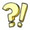There are two advantages to using implied odds and our Bet Calculators. First, it helps you determine if a betting market is beneficial, comprehending the meaning of VIG. Before we see in-depth how these prices work, let’s look at the rolling dice theory. It’s a theory that purely expresses (i.e., without margins etc.) how possibilities are presented in percentages. Rolling a dice yields a chance of one out of six for each outcome. The probability of the dice coming down on any of these six numbers is equal. That means that the implied probability of any of the six dice numbers to come up is 16,66%.

### How to calculate implied probability in decimal odds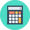When online betting sites set the fixed odds, they present a probability of each possible outcome in odds. These prices determine each result’s chances of happening, with decimal being the most common format type. You can manually convert them to implied odds solving the equation Implied Probability = (1/Decimal Odds) x 100. Let’s break out an example based on basketball and the NBA match between Miami Heat and Golden State Warriors. Read on the table below to see how the implied probability formula works.

 Heat vs. Warriors Decimal Odds Implied Probability (%) Heat to WIN 1.71 1/1.71 x 100 = 58,4795% Warriors to WIN 2.20 1/2.20 x 100 = 45,4545%

Converting the odds to probability on the NBA match, you realize that these numbers surpass 100%. That’s the bookmaker’s guaranteed profit margin. In our example, the VIG (i.e., 3,934%) isn’t so high, typical for 2-way markets. Using our Odds Converter, you can work out all odds formats and their implied probability.

#### How to work out decimal odds to percentage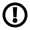Decimal odds express the most direct reflection of your possible outcome for each bet placed. Decimals of 2.00 odds, for example, mean that for every €1 you bet on a specific selection, you will receive a €1 as a payout. The implied probability of 2.00 odds is 50%. The table below presents how to read and convert the most common decimal odds to percentage, creating an odds archive based on their winning chances.

 Decimal Odds Implied Probability (%) 1.25 80% 1.50 66,7% 2.00 50% 2.50 40% 3.00 33,33% 3.50 28,6% 4.00 25% 5.00 20% 10.00 10% 100.00 1%

### How to convert fractional odds to probability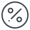The different odds formats are just another way to express the same thing, but the method to convert odds to probability isn’t the same. Let’s see how we can turn fractional betting odds into percentages using the English Premier League example. The table below contains the results from the implied probability formula {i.e., denominator / (denominator + numerator) x 100}, based on the match Leicester vs. Manchester City.

 Leicester vs. Manchester City Fractional Odds Implied Probability (%) Leicester to WIN 5/1 1/(1+5) x 100 = 16,6666% Draw 16/5 5/(5+16) x 100 = 23,8095% Manchester City to WIN 8/15 15/(15+8) x 100 = 65,2173%

The conclusions from this conversion are the great chances of City to win and the high commission. Compared to the example above, you can see that the margin here is considerably bigger at 5,6934%. In general, the more possible outcomes, the higher the VIG is.

### How to calculate implied odds from Moneylines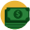There’s no difference in converting American odds from 3-way odds, but the two instances require solving two separate equations. To convert positive Moneyline odds to implied odds, use the mathematical equation 100/(Positive American Odds + 100) x 100. On the other hand, to convert negative American odds, you must solve the implied probability formula Negative American Odds/(Negative American Odds + 100) x 100. Let’s examine how to calculate implied odds with an example from the MLB match Colorado Rockies vs. Los Angeles Dodgers.

 Colorado Rockies vs. LA Dodgers Moneyline Odds Implied Probability (%) Rockies to WIN +185 100/(185+100) x 100 = 35,08% Dodgers to WIN -225 225/(225 + 100) x 100 = 69,23%

## Is implied probability the same as odds volatility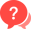These two terms should not be confused as they bear no resemblance whatsoever. Implied probability is the basis for creating an odds set or calculating the chances of a possible outcome. On the other hand, odds volatility represents how susceptible a specific set of odds is to price changes. Odds with high volatility will become shorter or longer with relative ease if large stakes suddenly back them. Volatility is mainly found in exchange markets from betting exchanges, stock exchanges and spreads betting. You can only take advantage of volatile odds by predicting odds movements in low-staked markets to place Sure Bets or cash out your profits.

### Why use implied probability odds in sports betting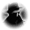Implied odds are a valuable alternative to read and understand the betting odds, which helps you answer the fundamental question, “how many chances will I have to win?” Using implied probability odds, you can find each selection’s value and discover how much you pay as a commission. The total payout is always less than what the bettors would receive if the odds reflected the actual chances. So, these odds are crucial for 1) finding value in betting, 2) choosing the best odds bookmakers, 3) estimating the likelihood of a possible outcome, and 4) securing the best prices.

You can simplify this process using our Implied Odds Calculator or manually convert them by reading our guide. Of course, this isn’t the only way because you can estimate a probable selection by using statistics or tactical analysis, depending on which sports you place a bet on. The implied probability odds helps you to find and take advantage of juicy odds with low VIG. Furthermore, considering the whole conversion process consistently, your chances to bet on value wagers and make a profit will ultimately increase.

Best Bookmakers for Sports Betting

You can find the best online betting sites that offer the most competitive odds and provide a wide range of markets and sports in the list underneath.

FAQ
Q: How to calculate fixed odds from implied odds?

There’s a specific equation that calculates the odds based on implied probability (%). For example, you can manually solve and learn the statistical correspondence for an implied probability at 40%; Odds = 1/Implied Probability = 1/(40/100) = 1/0.4 = 2.50.

Q: How to convert 21/20 odds into implied probability?

Using our Odds Converter you can instantly see that 21/20 odds means a 48,78% implied probability. If you want to make the conversion manually follow the equation 20/(20+21) x 100 = 20/41 x 100 = 0.4878 x 100 = 48,78%.

Q: How to convert 10 to 3 odds into implied odds?

Converting 10 to 3 odds with the implied probability formula the result is 3/(10+3) x 100 = 3/13 x 100 = 23.08. That means 23,08% implied odds and is translated at 4.33 decimals and +333 American odds.

Q: Is it easy to calculate implied probability on Hong Kong, Indonesian, or Malay odds?

If you use our Implied Odds Calculator or converter, it’s a matter of seconds to work out the Asian odds types with implied odds. Manually, the most accurate way is to convert Honk Kong, Indo, or Malay odds first to decimals, fractional or American odds and then calculate the implied probability.

Q: Why use betting instead of implied odds?

Betting odds is an alternate way to express implied probability and compare how much larger or smaller one chance is relative to another. A sporting event, for example, with a probability of 75% has 75 to 25 odds. The simplified format is 3/1, which means that one possibility is three times more likely to happen than the other.

Join and grab exclusive bonuses, daily offers and free bets!

Lay hands on exclusive daily bonuses for members only. These promos are limited and are not advertised on the website.

Yes, I have read the Terms & Conditions and I consent to receiving emails from Bookmakers.bet regarding betting promotions, betting guides and news.